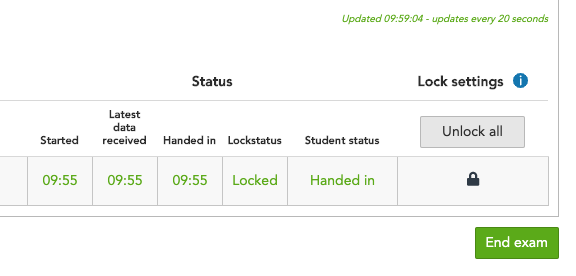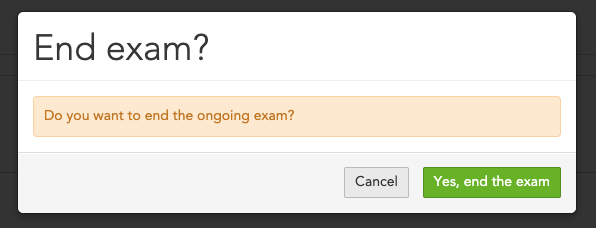Follow

# Ending an exam

After all students have handed in, the instructor needs to end the exam before they can begin grading.

Note! Before you end an exam, make sure that your students have handed in their answers first. If only one student started the exam, the exam will be erased if you end it before the student hand in their answers.

1. Click the green End exam button (see figure 1).Figure 1

2. Select the green Yes, end the exam button to end the exam (see figure 2).Figure 2

3. Your exam will be moved to the Ungraded tab (see figure 3). If the exams max point is set to 0 points the exam will be moved to Graded.Figure 3